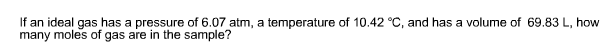# Problem: If an ideal gas has a pressure of 6.07 atm, a temperature of 10.42 °C, and has a volume of 69.83 L how many moles of gas are in the sample?

###### FREE Expert Solution

Using the ideal gas law: PV=nRT

where P is 6.07 atm, V is 69.83 L, T as 10.42oC and R as 0.0821

97% (382 ratings)###### Problem Details

If an ideal gas has a pressure of 6.07 atm, a temperature of 10.42 °C, and has a volume of 69.83 L how many moles of gas are in the sample?